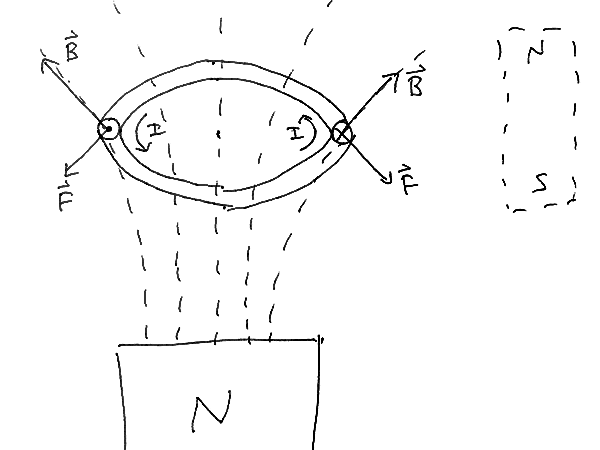# Explaining Magnetic Force on Iron Without Moving Charge

• Christopher R

#### Christopher R

Hi, I am a high school physics student and the only ways we learned to calculate the magnitude of a magnetic force involved a moving charge or current in a wire. As a result I have wondered how a bar magnetic magnet may exert a force on a piece of iron, since there is no clear movement of charge on the iron. If anyone here could explain this that would be amazing, although I fear it's probably going to be some complicated stuff with electrons. :)

Welcome to Physics Forums!

Ferromagnetism is the magic word.Go to

https://en.wikipedia.org/wiki/Ferromagnetism

and scroll down to the section Explanation. (Origin of magnetism, etc.) That should get you started. When you get stuck, feel free to ask questions here and someone can probably get you unstuck.

I sort of understand how the domains can allign and create a net magnetic field, but I'm still not sure how the actual attraction occurs. Like when the north end of a magnet is attracted to the south end of another, the direction of the magnetic field is on the same line as the direction of the force, but I thought that the resulting force is always perpindicular to the magnetic field. Is the force attracting magnets just fundamentally different than the magnetic force on moving charges?

There's two ways of looking at it. You can treat a magnet as if it has a current flowing around it, like a solenoid. Then it has moving charges, and the magnetic field exerts a force on these moving charges. But, I think this is kind of an ad hoc way of dealing with it. The other way is to just say that magnetic fields exert a force on magnetic dipoles. This force comes from the expression for magnetic energy.
##U=\frac{1}{2} \frac{B^2}{\mu}##
If the system has a spatial degree of freedom which allows the energy to decrease, then you have a force along that direction. If you try to force two north poles of two magnets together, you are increasing the magnetic energy near the north poles, so there is a force pushing them apart. In contrast, if you put a north and south pole together, the fields cancel and the magnetic energy is reduced.

Your energy explanation makes sense, but I am more confused by the actual mechanisms which cause there to be a force. If magnets are treated like solenoids, it still seems to me like there would be no net force between two magnets since the field would be pointed along the solenoid.

A Newtonian forced-based description is not necessarily any more fundamental than a Lagrangian or Hamiltonian energy-based description. Both give the "actual mechanics". But you can write the force of an external field on a dipole:
##F = \nabla (\mathbf{\mu} \cdot \mathbf{B})##
where ##\mu## is the dipole moment.

I'm still not sure how the actual attraction occurs. Like when the north end of a magnet is attracted to the south end of another, the direction of the magnetic field is on the same line as the direction of the force, but I thought that the resulting force is always perpindicular to the magnetic field.

To make it easier to visualize, replace one of the magnets with a loop of wire carrying a current, which behaves as a magnetic dipole very much like the magnet. The "north" side of the loop is in the direction given by the right-hand rule, upwards in this example. In the diagram, the plane of the loop is perpendicular to the axis of the magnet, and arrows indicate the current direction. At the left side of the loop, the current is towards you (perpendicular to your screen), and at the right side, it's away from you.

The magnetic field from the magnet spreads out, so it's at an angle to the plane of the loop. Therefore the magnetic force on each point of the loop is also at an angle. The net force on the loop is downward, i.e. the loop is attracted towards the magnet.Thanks jtbell, exactly the explanation i was looking for :D